# System subject to a driving force

• LCSphysicist

#### LCSphysicist

Homework Statement
All below
Relevant Equations
All below
The displacement of the end of a spring varies as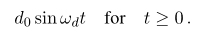, the block on the spring is subject to a viscous force proportional to the velocity. Spring stiffness k.

(a)Find the displacement of the block:
(b)When γ -> 0

First of all, i have a doubt i we could start saying the component of the force is the imaginary component, seems plausible?

Anyway:

## x'' + yx' + wo²*x = wo²*d*sin(wt) ##
## sin(wt) = cos(wt + 3pi/2) ## , x is Real part of z
## z'' + yz' + wo²z = wo²*d*e^{(wt + 3pi/2)i} ##
## Solving for z = A*e^{i(wt + 3pi/2)) ##

(a)X=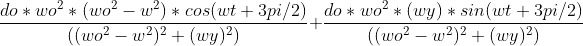+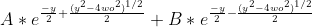(b)X =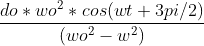+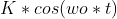That's ok?

#### Attachments

Last edited:

You mention a parameter ##y##. But you have not defined it. Presumably you mean for it to denote the viscosity. Naturally, this viscosity will be positive.

You mention a parameter ##o## which is always used as ##o^2##. Plausibly you mean for this to denote the spring constant k.

You use a parameter ##d##. But the parameter that is defined is ##d_0##.

You use a parameter ##\omega##. But the parameter that is defined is ##\omega_d##.

Can you rewrite your first equation after having defined the variables it uses?

•etotheipi

Being a body subject to a driven force F, in a medium whose constant of viscosity (T^-1) is y, and being x the displacement of the body/system.
That does not match the problem statement in the original post. There the driver was at a specified position rather than delivering a specified force.

Can we have an accurate problem statement, please?

•LCSphysicist
That does not match the problem statement in the original post. There the driver was at a specified position rather than delivering a specified force.

Can we have an accurate problem statement, please?
i tried to do the post in the cellphone, but made a huge confusion, it translate the message to my language, sorry. i deleted it. Anyway, now i think is better:

The block is attached to a cord that runs
over a pulley and is attached to a spring, as shown
The displacement of the end of the spring, subject to a driving force, varies as
## d*sin(w*t) ##
## t>=0 ##
and the block, which is attached to the spring, is subject to a viscous force proportional to the velocity, Fs.

## Fs = -m*y*v ##
Where v is the velocity of the body
and y is the damping coefficient [T^´-1]
m is the body mass.
and k is the spring stiffness
"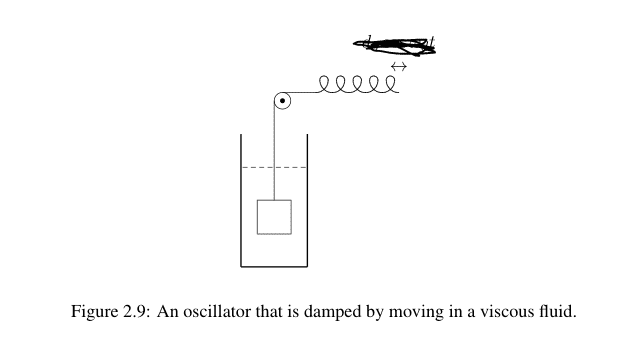(a)Find the displacement of the block:
(b)When γ -> 0​

#### Attachments

In your first equation, I don't understand the ##\omega_0^2x## term. Shouldn’t there be a k in there somewhere?

In your first equation, I don't understand the ##\omega_0^2x## term. Shouldn’t there be a k in there somewhere?
Yes, there is k, but i assumed that k = m*wo², where wo is the natural frequency. I though it would be more interesting an answer in function of wo.

Yes, there is k, but i assumed that k = m*wo², where wo is the natural frequency. I though it would be more interesting an answer in function of wo.
Ok, but it would have helped if you had defined ##\omega_0##, which you wrote as ##\omega o##. @jbriggs444 thought o was some other variable, and I assumed ##\omega_0## was a renaming of ##\omega_d##.
Is y supposed to be ##\gamma##?

I haven't checked the last parts of your solution, but I am a bit puzzled as to what happens when ##\gamma<2\omega_0##.

Perhaps someone can explain to me the setup in #4. As far as I can tell, if we define the coordinate ##y## of the block as the distance below the horizontal level of the spring, then our equation of motion is$$mg - T - c\dot{y} = m\ddot{y}$$Now if we define another coordinate ##x## which is the horizontal distance of the end of the spring from the peg, that$$x = d + d_0\sin{\omega_d t}$$where ##d## is the average ##x## coordinate of the end of the spring. Then the total length of the spring at any given time is (assuming the whole wire is the spring, it shouldn't matter),$$L(t) = y + d + d_0\sin{\omega t}$$The force ##T## must then be $$T = k\delta(t) = k(L(t) - L_0) = k(y + d + d_0\sin{\omega t} - L_0)$$and finally our equation of motion is$$mg - k(d-L_0) - kd_0\sin{\omega t} = m\ddot{y} + c\dot{y} + ky$$Have I got that right, or did I miss something?

If we were going to include gravity, we might consider buoyancy as well. But I see no need to include either. They are both equivalent to a fixed external force. Since we already have a spring in the problem, a fixed external force is equivalent to a change in the starting position.

We are not told the relaxed length of the spring. So we may as well do ourselves a favor and assume that the problem starts with the body at rest in its equilibrium position before the driver starts driving the spring.

•etotheipi
If we were going to include gravity, we might consider buoyancy as well. But I see no need to include either. They are both equivalent to a fixed external force. Since we already have a spring in the problem, a fixed external force is equivalent to a change in the starting position.

We are not told the relaxed length of the spring. So we may as well do ourselves a favor and assume that the problem starts with the body at rest in its equilibrium position before the driver starts driving the spring.

Thanks, yes I forgot about buoyancy. So I will recast the equation in terms of a constant force plus that due to the tension$$f - T = m\ddot{y} + c\dot{y}$$How to deal with the term ##T## in a simple manner still does not seem obvious to me. In my original setup$$T = k(y + d + d_0\sin{\omega t} - l_0)$$maybe you would like to express$$\dot{T} = k\dot{y} + d\omega \cos{\omega t}$$and insert$$-k\dot{y} - d\omega \cos{\omega t} = m\dddot{y} + c\ddot{y}$$ $$m\dddot{y} + c\ddot{y} + k\dot{y} = - d \omega \cos{\omega t}$$which is now a third order equation which should still yield to a standard constant coefficients method of solution...

Last edited by a moderator:
Ok, but it would have helped if you had defined ##\omega_0##, which you wrote as ##\omega o##. @jbriggs444 thought o was some other variable, and I assumed ##\omega_0## was a renaming of ##\omega_d##.
Is y supposed to be ##\gamma##?

I haven't checked the last parts of your solution, but I am a bit puzzled as to what happens when ##\gamma<2\omega_0##.
Well, if this happens, we are going to complex solutions and i think we would need to get the real parts, so the therms with euler would convert to something like
##y = K*e^(-yt/2)*cos(w1t + α)) ##

w1² = ##\omega_0^2 + y^2/4##)
About the problems of confusing the terms, i am still learning latex, actually i wrote all the equations in latex, but i probably made an error and so i prefer to write this in any program. Sorry

Actually i think all we hoping that this occurs
"I haven't checked the last parts of your solution, but I am a bit puzzled as to what happens when ##\gamma<2\omega_0##."
Otherwise, the motion wouldn't be oscillation, right?

@LCSphysicist I think you are on track for the differential equation you wrote down in the OP, but AFAIK that doesn't describe the scenario given in the question?

@LCSphysicist I think you are on track for the differential equation you wrote down in the OP, but AFAIK that doesn't describe the scenario given in the question?
Sorry... what is AFAIK?

Sorry... what is AFAIK?

Oh, it's just "as far as I know". I got that$$m\dddot{y} + c\ddot{y} + k\dot{y} = - d_0 \omega_d \cos{\omega_d t}$$

•LCSphysicist